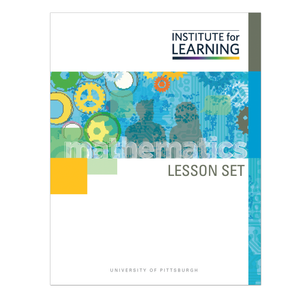# Solving Linear Equations in One Variable (Grade 8)

Regular price \$6.25 \$0.00 Unit price per

This lesson set provides students with the opportunity to write and solve linear equations in one variable, focusing particular attention on what it means for a value to be in the solution set of a linear equation in one variable.

Through engaging in this lesson set, students will:

• determine whether or not a value is in the solution set of a linear equation in one variable;
• use the distributive property, collecting like terms, and properties of equality to simplify and solve linear equations in one variable; and
• classify linear equations as having no solution, a unique solution, or infinitely many solutions.

Standards: 8.EE.C.7, 8.EE.C.7.A, 8.EE.C.7.B